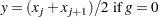Percentile Definitions

You can use the PCTLDEF= option to specify one of five definitions for computing quantile statistics (percentiles). Suppose thatis the number of nonmissing values for a variable and that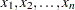represent the ordered values of the analysis variable. For the tth percentile, set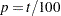.

For the following definitions numbered 1, 2, 3, and 5, express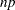as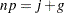whereis the integer part of, andis the fractional part of. For definition 4, let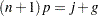The tth percentile (call it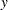) can be defined as follows:

PCTLDEF=1

weighted average at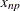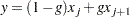where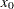is taken to be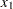.

PCTLDEF=2

observation numbered closest to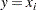whereis the integer part of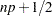if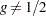. If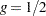, then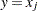ifis even, or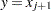ifis odd.

PCTLDEF=3

empirical distribution function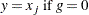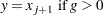PCTLDEF=4

weighted average aimed at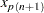where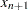is taken to be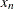.

PCTLDEF=5

empirical distribution function with averaging